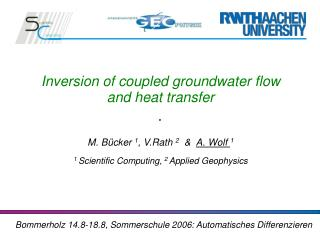Download PresentationInversion of coupled groundwater flow and heat transfer M. Bücker 1 , V.Rath 2 & A. Wolf 1

# Inversion of coupled groundwater flow and heat transfer M. Bücker 1 , V.Rath 2 & A. Wolf 1 - PowerPoint PPT PresentationDownload Presentation## Inversion of coupled groundwater flow and heat transfer M. Bücker 1 , V.Rath 2 & A. Wolf 1

- - - - - - - - - - - - - - - - - - - - - - - - - - - E N D - - - - - - - - - - - - - - - - - - - - - - - - - - -
##### Presentation Transcript

1. Inversion of coupled groundwater flow and heat transfer M. Bücker 1, V.Rath 2 & A. Wolf 1 1 Scientific Computing, 2 Applied Geophysics Bommerholz 14.8-18.8, Sommerschule 2006: Automatisches Differenzieren

2. Contents • Geothermal modeling in SHEMAT • Bayesian inversion method • Validation of the inversion code • Analytical solution • Numerical experiments • Covariance and Resolution matrices • Quality Indicator • Summary

3. SHEMAT equations FD solution of 3-D steady-state coupled fluid flow and heat transfer: Data h : hydraulic heads T : temperatures Parameter k : hydraulic permeability λ : thermal conductivity Others v : filtration velocity depending on hydraulic head Q, A : sources …

4. SHEMAT intern • Dirichlet and Neumann boundary conditions • fluid and rock properties dependent on temperature and pressure • nonlinear solution by simple alternating fixed point iteration • linear solvers • direct (from LAPACK) and iterative solvers (BiCGstab, parallelized with OpenMP )

5. SHEMAT in action

6. Data from Boreholes: temperatures and hydraulic heads Parameters: e.g. permeability, thermal conductivity. Underground structure sometimes well known, but measurements of parameters values often inadequate Questions: Is it possible to distinguish between advective and conductive effects? Which uncertainties will be present in the estimated parameters? Which data are necessary to constrain the estimate? Inverse geothermal modeling forward modeling, “SHEMAT” Inverse modeling, “SHEM_AD”

7. Parameterisation

8. General assumptions: A-priori error bounds of data and parameters Arbitrary integration of boundary conditions No ad-hoc regularisation parameters Bayesian Inversion Thomas Bayes, 1702-1761

9. Bayesian Estimation with: covariance matrices a priori residual Differentiate with respect to p, and apply Gauss-Newton method  with Jacobian • parameter covariance a posteriori

10. Analytical solution for coupled flow and heat transport Validation by analytical solution Péclet Number

11. Synthetic Models

12. Synthetic Models Free Convection(driven by density differences) Forced Convection(driven by surface topography ) T h

13. Temperature Sensitivities Free Convection Forced Convection +boundary conditions

14. Hydraulic Head Sensitivities Free Convection Forced Convection

15. Numerical experiment: type 1 Boreholes Reference model • Generate original data from initialisation parameters Goal • Estimate parameters Test setup • 8 data sets / runs • 8 boreholes chosen at random (position and depth) • consisting of temperatures, heads, or both • Data errors: ΔT = 0.5 K, Δh = 0.5 m • Error bars:

16. Parameter Fit units

17. units units Numerical experiment: data types

18. Numerical experiment (II) units units Forced Convection Free Convection

19. Numerical experiment: type 2 Boreholes Reference model • Same original data from initialisation parameters Goal • Estimate parameters Test setup • 8 data sets / runs • consisting of4, 8, or12 boreholes • each chosen at random (position and depth) • Data errors: ΔT = 0.5 K, Δh = 0.5 m • Error bars:

20. Synthetic Models: how many data? units units

21. Data Fit: Forced Convection • One of the runs with 8 randomly chosen boreholes • Temperature and head data • Inversion converged • Adequate parameters estimated

22. Information Discussion Questions: • Which uncertainties will be present in the estimated parameters? • Which data are necessary to constrain the estimate?

23. Covariance a posteriori Thermal Conductivity Permeability units units units Covariance matrix a posteriori near the minimum of θB: • Disadvantage: full Jacobian matrix

24. Resolution matrices Parameter resolution matrix (solution inverse problem) Free Convection Thermal Conductivity units units

25. Experimental Design: 3-D Model Model (14 zones) Permeability Temperature

26. Design Quality Indicators “Generalized Inverse” M M • Top view • Sensitivities for 0 to 2000m • Advantage: row compressed Jacobian matrix

27. Parameter Sensitivity Matrix

28. Summary and Conclusions • Successful validation without data from real experiments, which can be expensive • Covariance and Resolution matrices can help to decide which parameters needs to be determent more exactly • But their computation may be expensive • Future work: • Reverse-Mode AD version make it possible to use algorithms with: • improved convergency (matrix free Newton-Krylow) • smaller memory requirements for larger models • Validation with real experimental data

29. The End THANK YOU FOR YOUR ATTENTION !